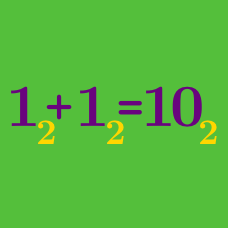Number Theory

# Number Bases: Level 5 ChallengesThe first expedition to a distant planet found only the ruins of a civilization. The explorers were able to translate the "extraterrestrial" equation they found there as follows:

$5x^2 -50x +125 = 0. \text{ Therefore, } x \text{ is equal to } 5 \text{ or } 8.$

This was an extremely strange result. The value $x=5$ seemed satisfactory, but $x=8$ required some explanation. If their number system were similar to ours, how many fingers would you say the inhabitants in that planet had?

Make the assumption that if the inhabitants had $n$ fingers, then they work in base $n$.

What is the 50th smallest positive integer that can be written as the sum of one or more distinct powers of 3 with non-negative integer exponents?

In the infinite series $\dfrac{1}{9}+\dfrac{1}{99}+\cdots + \dfrac{1}{10^n-1}+\cdots$at what place after the decimal point does the second $1$ occur?

$\text{Details and Assumptions}$

The first $1$ occurs at the first place after the decimal point.

Doge has become the king of a huge empire and wants to show the world that he is really intelligent. He is so over ambitious that his first action as king is to name the currency after himself: DogeCoin. He wants to issue $d$ denominations of coins so that using no more than 3 coins, citizens can pay any amount from 1 DogeCoin to 36 DogeCoin in exact change.

Find the minimum value of $d$ and all the corresponding denominations of DogeCoin. Submit your answer as the sum of these denominations.

Details and Assumptions:

• As an explicit example, if you get the denominations as $\{10, 12, 15\}$, then input the answer as $10+12+15=37$.

There exists a unique 10-digit number $\overline{abcdefghij}$ which contains each of the digits 0, 1, 2, $\dots$, 9 exactly once, such that for each $k$, $1 \le k \le 10$, the number formed by the first $k$ digits of $\overline{abcdefghij}$ is divisible by $k$. For example, for $k = 4$, the number $\overline{abcd}$ is divisible by 4.

Find the three-digit number $\overline{abc}$.

×